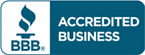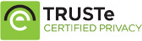# Test bank for Essential Calculus 2nd Edition by James Stewart

\$30.00

Essential Calculus 2nd Edition by James Stewart Test bank Questions

Full Chapters are includedCategories: ,

## Description

Test bank for Essential Calculus 2nd Edition by James Stewart

1. FUNCTIONS AND LIMITS.
Functions and Their Representations. A Catalog of Essential Functions. The Limit of a Function. Calculating Limits. Continuity. Limits Involving Infinity.
2. DERIVATIVES.
Derivatives and Rates of Change. The Derivative as a Function. Basic Differentiation Formulas. The Product and Quotient Rules. The Chain Rule. Implicit Differentiation. Related Rates. Linear Approximations and Differentials.
3. APPLICATIONS OF DIFFERENTIATION.
Maximum and Minimum Values. The Mean Value Theorem. Derivatives and the Shapes of Graphs. Curve Sketching. Optimization Problems. Newton’s Method. Antiderivatives.
4. INTEGRALS.
Areas and Distances. The Definite Integral. Evaluating Definite Integrals. The Fundamental Theorem of Calculus. The Substitution Rule.
5. INVERSE FUNCTIONS.
Inverse Functions. The Natural Logarithmic Function. The Natural Exponential Function. General Logarithmic and Exponential Functions. Exponential Growth and Decay. Inverse Trigonometric Functions. Hyperbolic Functions. Indeterminate Forms and l’Hospital’s Rule.
6. TECHNIQUES OF INTEGRATION.
Integration by Parts. Trigonometric Integrals and Substitutions. Partial Fractions. Integration with Tables and Computer Algebra Systems. Approximate Integration. Improper Integrals.
7. APPLICATIONS OF INTEGRATION.
Areas between Curves. Volumes. Volumes by Cylindrical Shells. Arc Length. Area of a Surface of Revolution. Applications to Physics and Engineering. Differential Equations.
8. SERIES.
Sequences. Series. The Integral and Comparison Tests. Other Convergence Tests. Power Series. Representing Functions as Power Series. Taylor and Maclaurin Series. Applications of Taylor Polynomials.
9. PARAMETRIC EQUATIONS AND POLAR COORDINATES.
Parametric Curves. Calculus with Parametric Curves. Polar Coordinates. Areas and Lengths in Polar Coordinates. Conic Sections in Polar Coordinates.
10. VECTORS AND THE GEOMETRY OF SPACE.
Three-Dimensional Coordinate Systems. Vectors. The Dot Product. The Cross Product. Equations of Lines and Planes. Cylinders and Quadric Surfaces. Vector Functions and Space Curves. Arc Length and Curvature. Motion in Space: Velocity and Acceleration.
11. PARTIAL DERIVATIVES.
Functions of Several Variables. Limits and Continuity. Partial Derivatives. Tangent Planes and Linear Approximations. The Chain Rule. Directional Derivatives and the Gradient Vector. Maximum and Minimum Values. Lagrange Multipliers.
12. MULTIPLE INTEGRALS.
Double Integrals over Rectangles. Double Integrals over General Regions. Double Integrals in Polar Coordinates. Applications of Double Integrals. Triple Integrals. Triple Integrals in Cylindrical Coordinates. Triple Integrals in Spherical Coordinates. Change of Variables in Multiple Integrals.
13. VECTOR CALCULUS.
Vector Fields. Line Integrals. The Fundamental Theorem for Line Integrals. Green’s Theorem. Curl and Divergence. Parametric Surfaces and Their Areas. Surface Integrals. Stokes’ Theorem. The Divergence Theorem.
Appendix A. Trigonometry.
Appendix B. Proofs.
Appendix C. Sigma Notation.

## Reviews

There are no reviews yet.